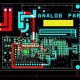# What are Topology & its types?

In this tutorial we are going to learn about what are Topology & its types?

Topology – The physical arrangement of the computer system/node, which is connected to each other via communication medium, is called topology.

Types of Topologies:

1. Bus Topology
2. Ring Topology
3. Star Topology
4. Mesh Topology
5. Hybrid Topology
6. Tree Topology
• Bus Topology – In bus topology, one long cable acts as a single communication channel & all the devices are connected to this cable.In this below diagram for Bus topology can see the there is showing bus topology configuration.
• Ring Topology – It is called ring topology because it forms a ring, in this topology each node is strongly connected with its adjacent node. In this below diagram for bus topology can see the there is showing Ring topology configuration.
• Star Topology – In star topology all the nodes are connected with a central device called HUB, and the sharing of data is only possible through HUB. In this below diagram for Star topology can see the there is showing bus topology configuration.

Mesh Topology – In this topology each and every computer is directly connected with each other, so we can directly send the data to the destination machine without going to intermediate machine. In this below diagram for bus topology can see the there is showing Mesh topology configuration.

• Hybrid Topology – It is combination of various different topologies is called hybrid topology. . In this below diagram for Hybrid topology can see the there is showing Mesh topology configuration.
• Tree Topology – In this topology, all the nodes are connected like a bus & star topology is called tree topology.  In this below diagram for bus topology can see the there is showing Tree topology configuration.